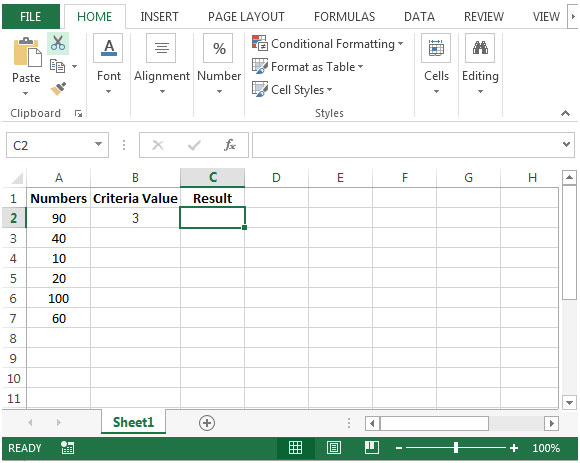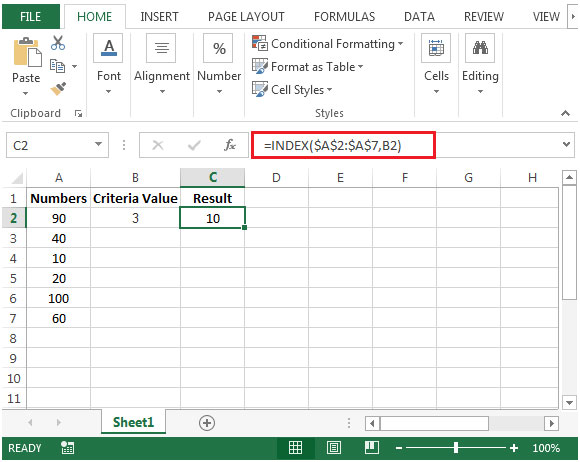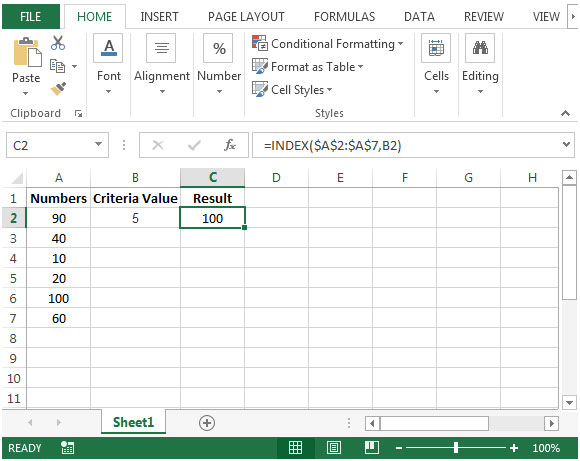# Retrieving from List by Position

To retrieve value from list by position, we will use INDEX function to get the output.

What is Index function and how we can use the Index function in Microsoft Excel?

INDEX: Returns a value or reference of the cell at the intersection of a particular row and column, in a given range.

Syntax: =INDEX(array,row_num,column_num)

array:Array is a range of cells or table.

row_num:The row number in the array from which the value is returned.

column_num:It is optional. It is the column number in the array that is used to return the value.

Let us take an example:

We have some random numbers in column A. Criteria is set in cell B2.We need a formula to lookup the criteria & extract the nth number of value from column A.• In cell C2, the formula would be =INDEX(\$A\$2:\$A\$7,B2)• If we change the value in cell B2 from 3 to say 5 then the formula will retrieve the result accordingly.This is the way we can use the Index function in Microsoft Excel.

Terms and Conditions of use

The applications/code on this site are distributed as is and without warranties or liability. In no event shall the owner of the copyrights, or the authors of the applications/code be liable for any loss of profit, any problems or any damage resulting from the use or evaluation of the applications/code.